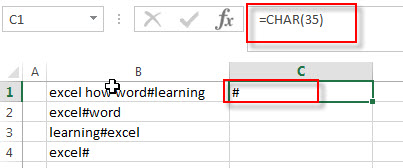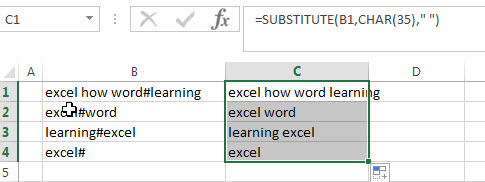# How to remove unwanted characters from text string

This post explains that how to remove unwanted characters from text string in a Cell in Excel. How do I remove unwanted characters from a cell using Excel formula.

## Remove Unwanted Characters

If you want to remove unwanted or specified characters from a text string, you can create an excel formula based on the SUBSTITUTE function and CHAR function.

You can use CHAR function get a character from a code number, then using SUBSTITUTE function to replace this character with empty string.

Assuming that you want to remove hash character from text string in Cell B1, then you can write down the following formula:

`=SUBSTITUTE(B1,CHAR(35),"")`

Let’s see how this formula works:

=CHAR(35)The CHAR function returns a character based on the given character code, and this function returns a hash character.

=SUBSTITUTE(B1,CHAR(35),” “)This formula will replace all hash character returned by the CHAR function with empty string, it means that it will remove all hash characters from text string in Cell B1.

You can refer to the below link for the character set:

### Related Functions

• Excel Substitute function
The Excel SUBSTITUTE function replaces a new text string for an old text string in a text string.The syntax of the SUBSTITUTE function is as below:= SUBSTITUTE  (text, old_text, new_text,[instance_num])….
• Excel CHAR function
The Excel CHAR function returns the character specified by a number (ASCII Value). The syntax of the CHAR function is as below: =CHAR(number)….